## Example Questions

### Example Question #1 : How To Find The Length Of The Side Of A Right Triangle

A right triangle's perimeter is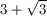. The other two angles of the triangle are 30 degrees and 60 degrees.

Quantity A: The triangle's hypotenuse length

Quantity B: 2

Possible Answers:

The two quantities are equal.

Quantity B is greater.

Quantity A is greater.

The relationship cannot be determined from the information given.

Correct answer:

The two quantities are equal.

Explanation:

The ratio of the sides of a 30-60-90 triangle is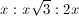, with the hypotenuse being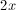. Thus, the perimeter of this triangle would be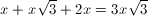. Since the triangle depicted in this problem has a perimeter of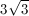must equal 1, which would make the hypotenuse equal to 2.

### Example Question #1 : How To Find The Length Of The Side Of A Right Triangle

If the shortest side of a right triangle has length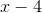and its hypotenuse has length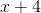, what is the length of the remaining side?

Possible Answers: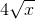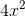Correct answer:Explanation:

Use the Pythagorean theorem,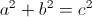, with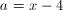and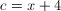, and solve for.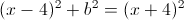Rearrange to isolate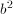: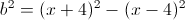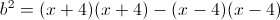Use FOIL to multiply out: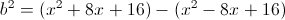Distribute the minus sign to rewrite without parentheses: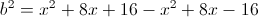Combine like terms: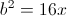Take the square root of both sides: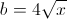### Example Question #1 : How To Find The Length Of The Side Of A Right Triangle

Given the following triangle, what is the length of the unknown side?Possible Answers:

The answer cannot be determined from the information given.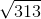Correct answer:

The answer cannot be determined from the information given.

Explanation:

At first sight, it's tempting to assume this is a right triangle and to thus use the Pythagorean Theorem to find a length of 5 for the missing side.

However, the triangle was not stated to be a right triangle in the problem statement, and no indication was given in the drawing to indicate that it was a right triangle either, such as a square demarcation in the vertex opposite the side measuring 13.

Thus there is not enough information to give the length of the missing side. When taking standardized math tests, be careful making assumptions about information that is not given.

### Example Question #1 : How To Find The Height Of A Right Triangle

An equilateral triangle has a side length of 4. What is its height?

Possible Answers:

2√2

2√3

4

16

2

Correct answer:

2√3

Explanation:

If an equilateral triangle is divided in 2, it forms two 30-60-90 triangles. Therefore, the side of the equilateral triangle is the same as the hypotenuse of a 30-60-90 triangle. The side lengths of a 30-60-90 triangle adhere to the ratio x: x√3 :2x. since we know the hypothesis is 4, we also know that the base is 2 and the height is  2√3.

### Example Question #2 : How To Find The Length Of The Hypotenuse Of A Right Triangle : Pythagorean Theorem

Which of these triangles cannot have a right angle?

Possible Answers:

9, 12, 15

5, 12, 13

6,7,12

2, 2, 2√2

√2, √2, 2

Correct answer:

6,7,12

Explanation:

6, 7, 12 cannot be the side lengths of a right triangle. 62+ 7does not equal 122. Also, special right triangle 3-4-5, 5-12-13, and 45-45-90 rules can eliminate all the other choices.

### Example Question #1 : How To Find The Length Of The Hypotenuse Of A Right Triangle : Pythagorean Theorem

Daria and Ashley start at the same spot and walk their two dogs to the park, taking different routes. Daria walks 1 mile north and then 1 mile east. Ashley walks her dog on a path going northeast that leads directly to the park. How much further does Daria walk than Ashley?

Possible Answers:

Cannot be determined

1 mile

2 + √2 miles

√2 miles

2 – √2 miles

Correct answer:

2 – √2 miles

Explanation:

First let's calculate how far Daria walks. This is simply 1 mile north + 1 mile east = 2 miles. Now let's calculate how far Ashley walks. We can think of this problem using a right triangle. The two legs of the triangle are the 1 mile north and 1 mile east, and Ashley's distance is the diagonal. Using the Pythagorean Theorem we calculate the diagonal as √(12 + 12) = √2. So Daria walked 2 miles, and Ashley walked √2 miles. Therefore the difference is simply 2 – √2 miles.

### Example Question #91 : Geometry

Which of the following sets of sides cannnot belong to a right triangle?

Possible Answers:

6, 7, 8

5, 12, 13

2, 2, 2√2

2, 2√3, 4

3, 4 ,5

Correct answer:

6, 7, 8

Explanation:

To answer this question without plugging all five answer choices in to the Pythagorean Theorem (which takes too long on the GRE), we can use special triangle formulas. Remember that 45-45-90 triangles have lengths of x, x, x√2.  Similarly, 30-60-90 triangles have lengths x, x√3, 2x.  We should also recall that 3,4,5 and 5,12,13 are special right triangles.  Therefore the set of sides that doesn't fit any of these rules is 6, 7, 8.

### Example Question #91 : Plane Geometry

Max starts at Point A and travels 6 miles north to Point B and then 4 miles east to Point C. What is the shortest distance from Point A to Point C?

Possible Answers:

10 miles

7 miles

2√13 miles

4√2 miles

5 miles

Correct answer:

2√13 miles

Explanation:

This can be solved with the Pythagorean Theorem.

62 + 42 = c2

52 = c2

c = √52 = 2√13

### Example Question #51 : Plane Geometry

Which set of side lengths CANNOT correspond to a right triangle?

Possible Answers:

7, 24, 25

3, 4, 5

5, 12, 13

8, 15, 17

6, 8, 11

Correct answer:

6, 8, 11

Explanation:

Because we are told this is a right triangle, we can use the Pythagorean Theorem, a2 + b2 = c2. You may also remember some of these as special right triangles that are good to memorize, such as 3, 4, 5.

Here, 6, 8, 11 will not be the sides to a right triangle because 62 + 82 = 102.

### Example Question #61 : Triangles

Kathy and Jill are travelling from their home to the same destination. Kathy travels due east and then after travelling 6 miles turns and travels 8 miles due north. Jill travels directly from her home to the destination. How miles does Jill travel?

Possible Answers: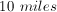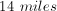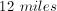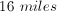Correct answer:Explanation:

Kathy's path traces the outline of a right triangle with legs of 6 and 8. By using the Pythagorean Theorem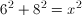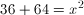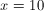miles

### All GRE Math Resources